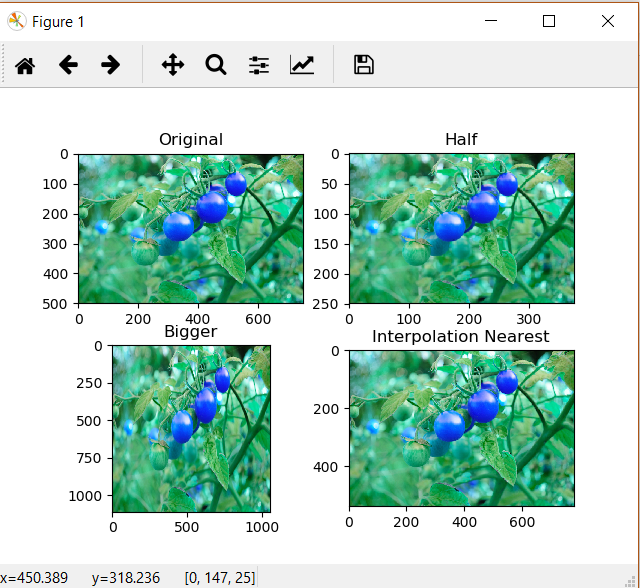Open in App
Not now

# Image Resizing using OpenCV | Python

• Difficulty Level : Easy
• Last Updated : 16 Jan, 2023

Image resizing refers to the scaling of images. Scaling comes in handy in many image processing as well as machine learning applications. It helps in reducing the number of pixels from an image and that has several advantages e.g. It can reduce the time of training of a neural network as the more the number of pixels in an image more is the number of input nodes that in turn increases the complexity of the model.
It also helps in zooming in on images. Many times we need to resize the image i.e. either shrink it or scale it up to meet the size requirements. OpenCV provides us several interpolation methods for resizing an image.

Choice of Interpolation Method for Resizing:

• cv2.INTER_AREA: This is used when we need to shrink an image.
• cv2.INTER_CUBIC: This is slow but more efficient.
• cv2.INTER_LINEAR: This is primarily used when zooming is required. This is the default interpolation technique in OpenCV.

Syntax: cv2.resize(source, dsize, dest, fx, fy, interpolation)

Parameters:

• source: Input Image array (Single-channel, 8-bit or floating-point)
• dsize: Size of the output array
• dest: Output array (Similar to the dimensions and type of Input image array) [optional]
• fx: Scale factor along the horizontal axis  [optional]
• fy: Scale factor along the vertical axis  [optional]
• interpolation: One of the above interpolation methods  [optional]

Below is the code for resizing:

## Python3

 `import` `cv2` `import` `numpy as np` `import` `matplotlib.pyplot as plt`   `image ``=` `cv2.imread(r``"D:\sims\eb\sim21\EB-ML-06-10-2022-Test-Output-15\PERFORATION\Overkill\Fail\Blister 1 2022-03-12 12-59-43.859 T0 M0 G0 3 PERFORATION Mono.bmp"``, ``1``)` `# Loading the image`   `half ``=` `cv2.resize(image, (``0``, ``0``), fx ``=` `0.1``, fy ``=` `0.1``)` `bigger ``=` `cv2.resize(image, (``1050``, ``1610``))`   `stretch_near ``=` `cv2.resize(image, (``780``, ``540``), ` `               ``interpolation ``=` `cv2.INTER_LINEAR)`     `Titles ``=``[``"Original"``, ``"Half"``, ``"Bigger"``, ``"Interpolation Nearest"``]` `images ``=``[image, half, bigger, stretch_near]` `count ``=` `4`   `for` `i ``in` `range``(count):` `    ``plt.subplot(``2``, ``2``, i ``+` `1``)` `    ``plt.title(Titles[i])` `    ``plt.imshow(images[i])`   `plt.show()`

Output:Note: One thing to keep in mind while using the cv2.resize() function is that the tuple passed for determining the size of the new image ((1050, 1610) in this case) follows the order (width, height) unlike as expected (height, width).

My Personal Notes arrow_drop_up
Related Articles